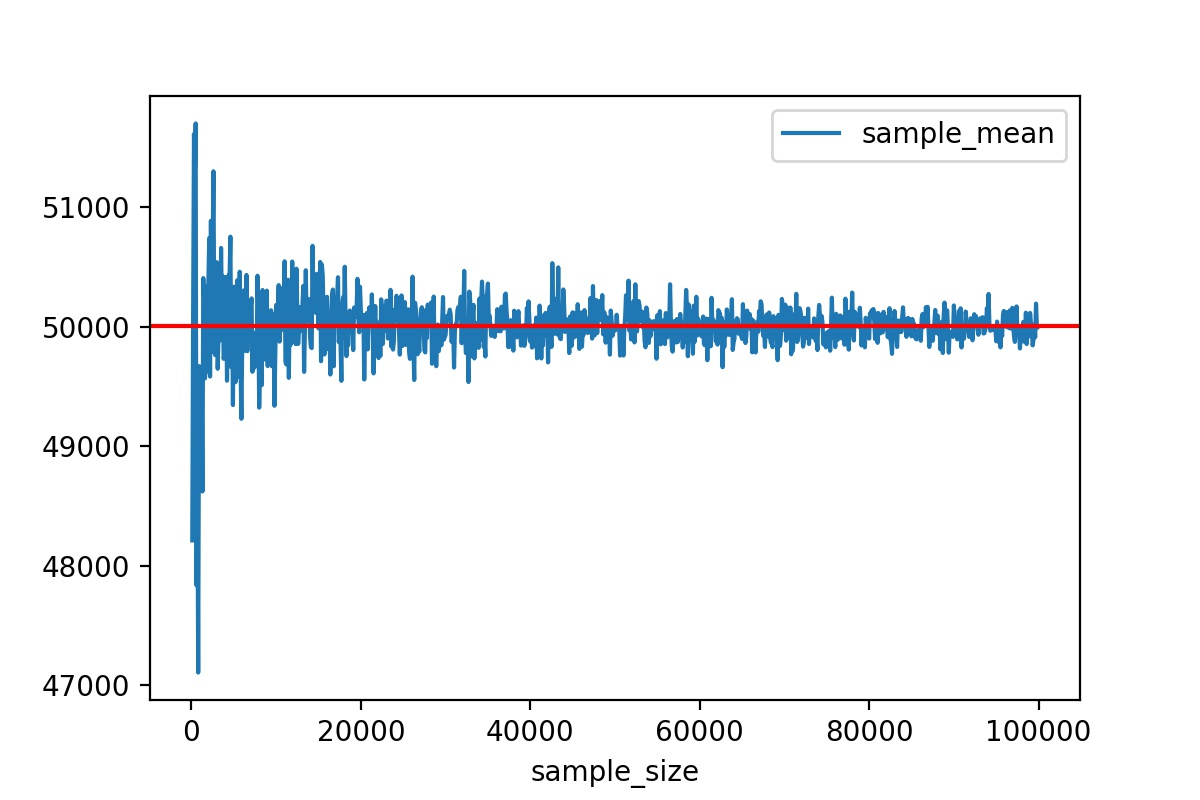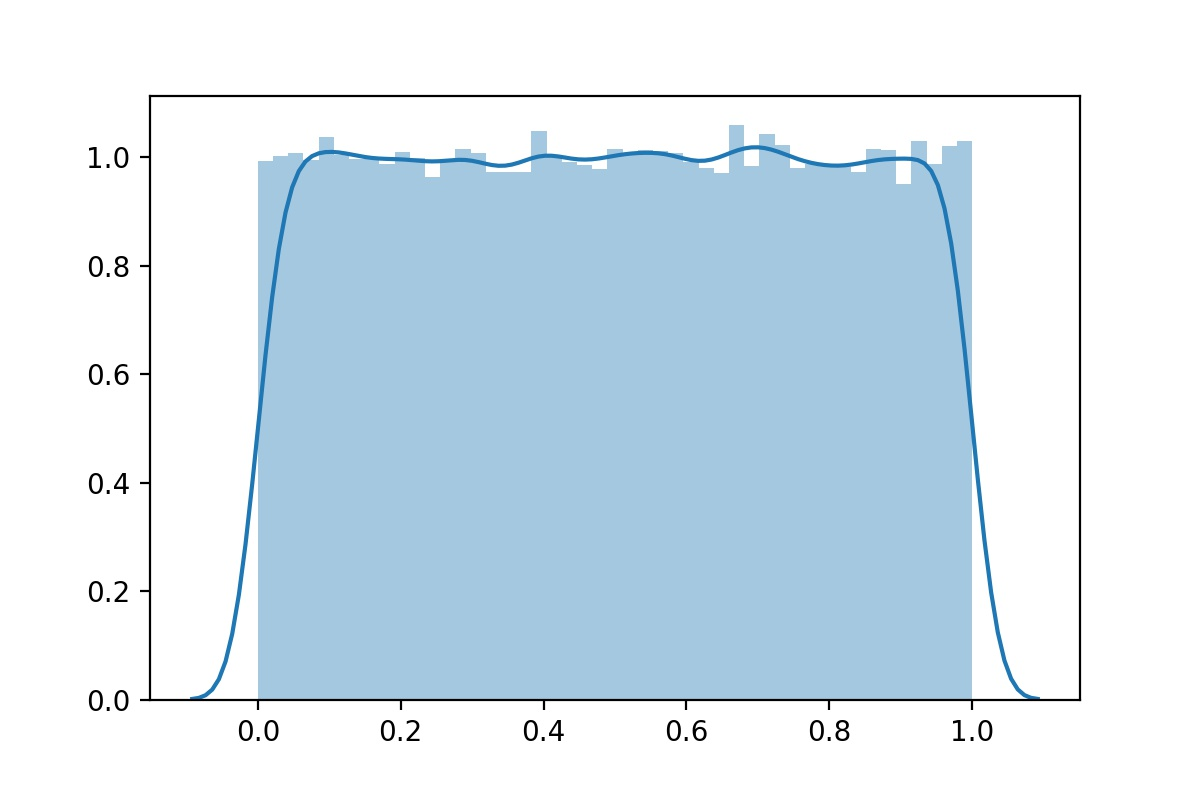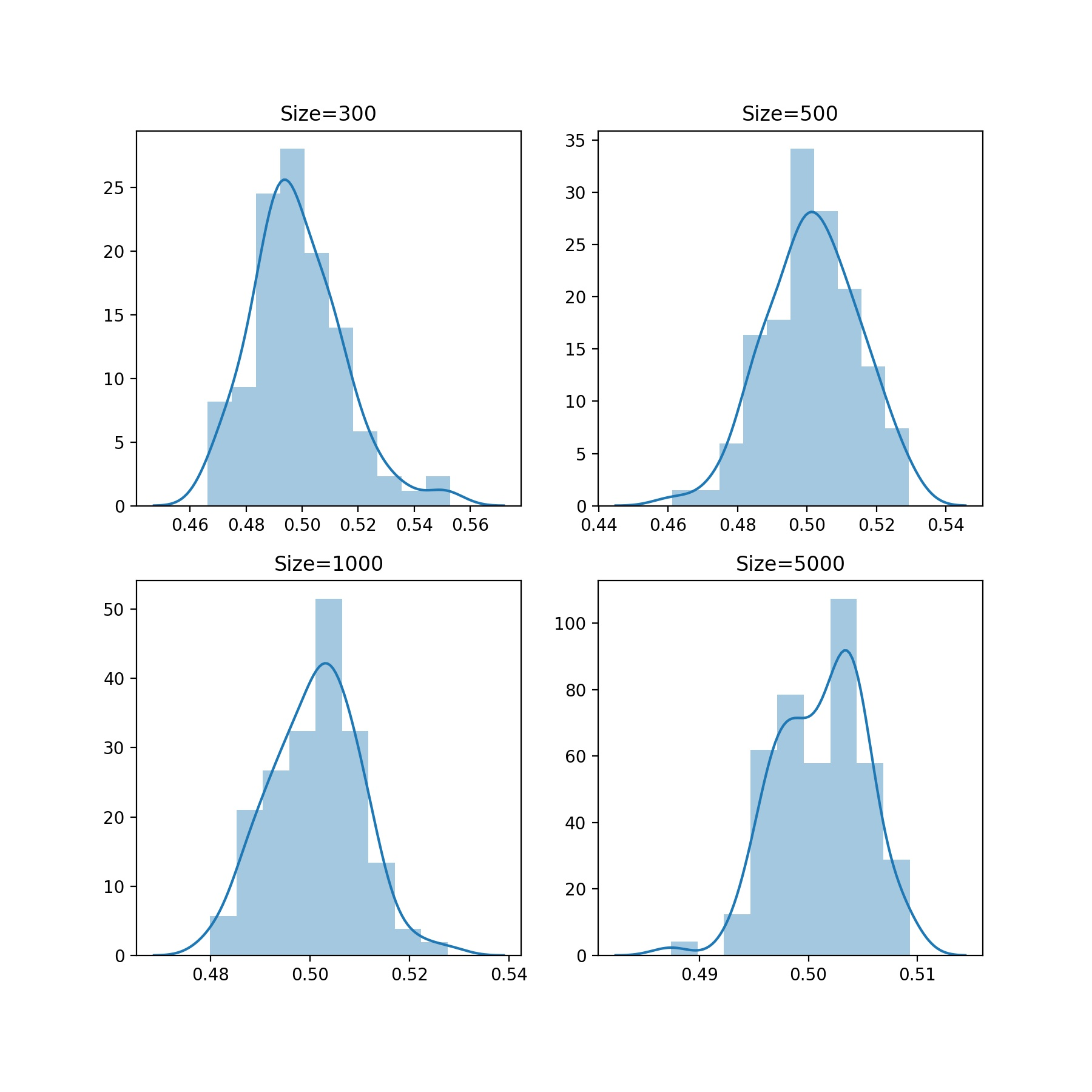# Python学习笔记：大数定理和中心极限定理

## 一、大数定理

###### 大数定理 ######
import os
import numpy as np
import pandas as pd
import matplotlib.pyplot as plt

os.chdir(r"C:\Users\Hider\Desktop")

data = np.random.randint(1, 100000, 100000)
# np.random.randint(low, high, size) 产生离散均匀分布的整数
sample_size = []
# sample_size = [i for i in range(100,100000,100)]
# 直接列表推导式生成效率更高
sample_mean = []

for i in range(100, 100000, 100):
sample_size.append(i)
sample_mean.append(np.random.choice(data, i).mean())

pd.DataFrame({"sample_size":sample_size,
"sample_mean":sample_mean}).set_index("sample_size").plot()
plt.axhline(data.mean(), color="red")
# 绘制平行于x轴的水平参考线
plt.savefig("大数定理.jpg", dpi=200)
plt.show() # 先保存再show## 二、中心极限定理

###### 中心极限定理 ######
import numpy as np
import pandas as pd
import seaborn as sns
import matplotlib.pyplot as plt

data = np.random.rand(100000)
sns.distplot(data)
plt.savefig("中心极限定理分布图1.jpg", dpi=200)
plt.show()# 重复抽取100次
# 创建画布
plt.figure(figsize = (9,9))
# 图1
plt.subplot(221)
sample_mean =[]
for i in range(1, 100):
s = np.random.choice(data, size=300).mean()
sample_mean.append(s)
sns.distplot(sample_mean)
plt.title("Size=300")
# 图2
plt.subplot(222)
sample_mean =[]
for i in range(1, 100):
s = np.random.choice(data, size=500).mean()
sample_mean.append(s)
sns.distplot(sample_mean)
plt.title("Size=500")
# 图3
plt.subplot(223)
sample_mean =[]
for i in range(1, 100):
s = np.random.choice(data, size=1000).mean()
sample_mean.append(s)
sns.distplot(sample_mean)
plt.title("Size=1000")
# 图4
plt.subplot(224)
sample_mean =[]
for i in range(1, 100):
s = np.random.choice(data, size=5000).mean()
sample_mean.append(s)
sns.distplot(sample_mean)
plt.title("Size=5000")
plt.savefig("中心极限定理分布图2.jpg", dpi=200)
plt.show()posted @ 2021-05-09 15:08  Hider1214  阅读(88)  评论(0编辑  收藏  举报